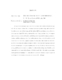## Two-dimensional Turbulence with Drag##### Files
umi-umd-1708.pdf(5.93 MB)
We consider the enstrophy cascade in forced two-dimensional turbulence with a linear drag force. In the presence of linear drag, the energy wavenumber spectrum drops with a power law faster than in the case without drag, and the vorticity field becomes intermittent, as shown by the anomalous scaling of the vorticity structure functions. Using a previous theory, we compare numerical simulation results with predictions for the power law exponent of the energy wavenumber spectrum and the scaling exponents of the vorticity structure functions $\zeta_{2q}$ obtained in terms of the distribution of finite time Lyapunov exponents. We also study, both by numerical experiment and theoretic al analysis, the multifractal structure of the viscous enstrophy dissipation in terms of its R\'{e}nyi dimension spectrum $D_q$ and singularity spectrum $f(\alpha)$. We derive a relation between $D_q$ and $\zeta_{2q}$, and discuss its relevance to a version of the refined similarity hypothesis. In addition, we obtain and compare theoretically and numerically derived results for the dependence on separation $r$ of the probability distribution of $\delta_{\V{r}}\omega$, the difference between the vorticity at two points separated by a distance $r$. Our numerical simulations are done on a $4096 \times 4096$ grid.